IntroductionIntroduction# Area of Triangles

INTRO
When we’re asked to find the area of a triangle, what we’re looking for is the amount of space inside the sides of our triangle.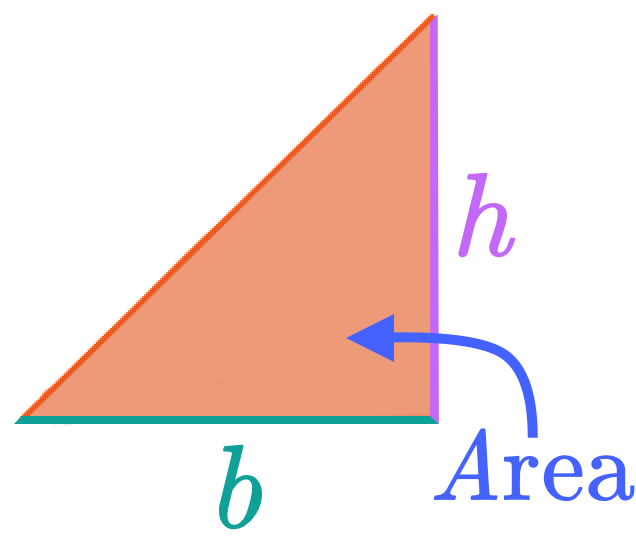The formula is pretty straightforward, but correctly identifying the base and the height of the triangle can sometimes be tricky. That’s why we like to imagine triangles as mountains!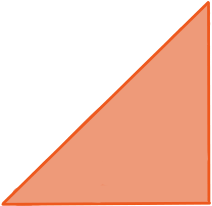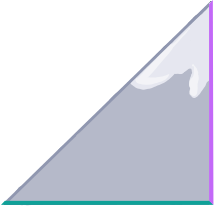### Type of Triangle:

We can imagine the triangle as a mountain where the base is flat (), and the height is a vertical line that connects the base to the peak ().
CALCULATOR

## Area of Triangles Calculator

What does your triangle look like?

#### Step 1. Identify the base and height of the triangle.

Imagine the triangle is a mountain. The base must be flat and the height connects the base of the mountain to the peak.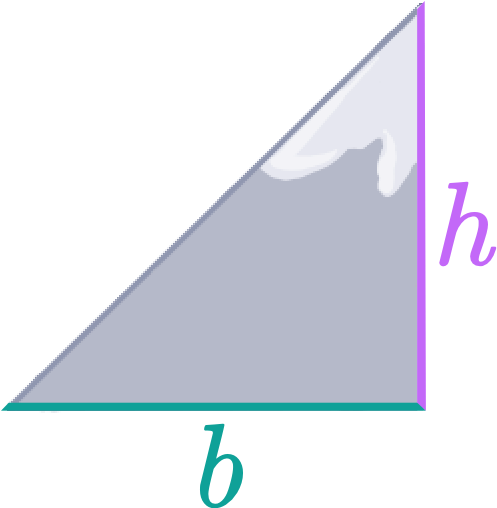KEY STEPS

## How to Find the Area of a Triangle

### Step 1. Identify the base and height of the triangle.

Imagine the triangle is a mountain. The base must be flat and the height connects the base of the mountain to the peak.### Step 3. Divide by 2.

We divide by 2 because the area of a right triangle is equal to ½ the area of a rectanglemade up of two right triangles with the same base and height.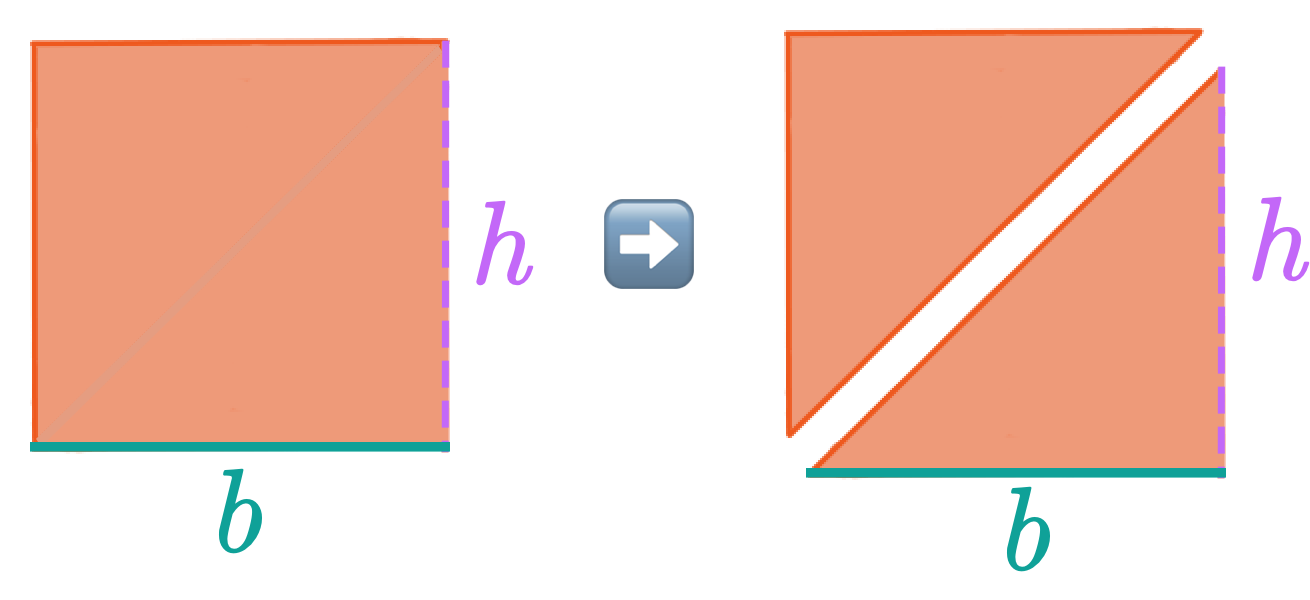LESSON
Right Triangles

## Right Triangles

The area of a right triangle is equal to the area of a rectangle made up of two right triangles with the same base and height:Let's do some practice problems!
PRACTICE
Right Triangles

## Practice: Area of Right Triangles

Question 1 of 3: Find the area of this triangle ⬇️### Step 1. Identify the base and height of the triangle.

Imagine the triangle is a mountain. The base must be flat and the height connects the base of the mountain to the peak.

LESSON
Acute Triangles

## Acute Triangles

The area of an acute triangle is equal to the area of a parallelogram made up of two acute triangles with the same base and height: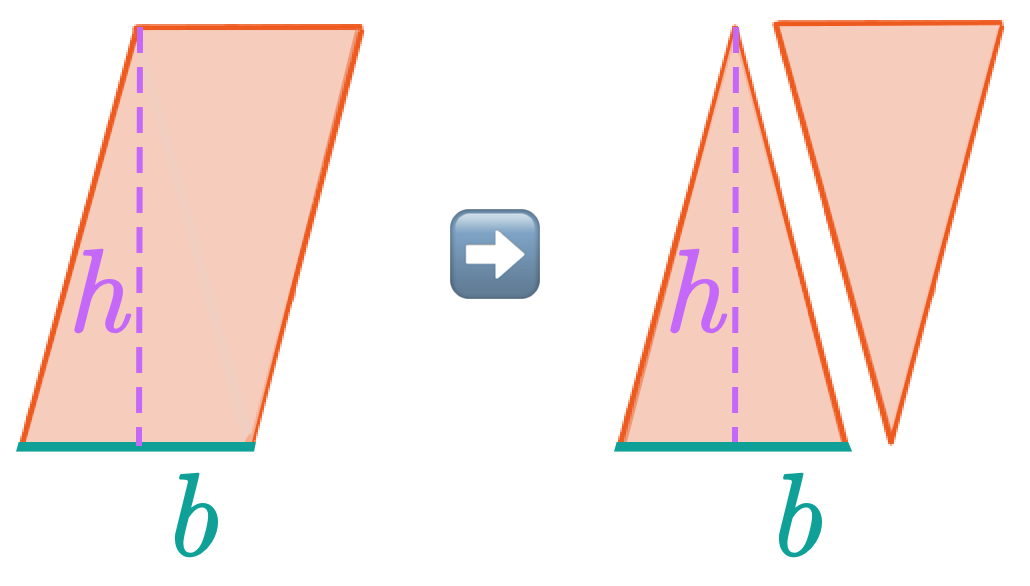PRACTICE
Acute Triangles

## Practice: Area of Acute Triangles

Question 1 of 3: Find the area of this triangle ⬇️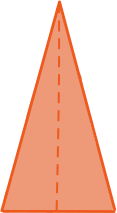### Step 1. Identify the base and height of the triangle.

Imagine the triangle is a mountain. The base must be flat and the height connects the base of the mountain to the peak.

LESSON
Obtuse Triangles

## Obtuse Triangles

The area of an obtuse triangle is equal to the area of a parallelogram made up of two obtuse triangles with the same base and height: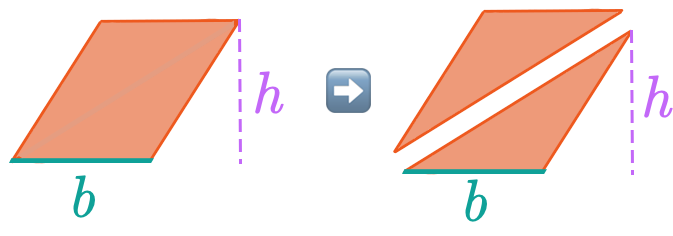PRACTICE
Obtuse Triangles

## Practice: Area of Obtuse Triangles

Question 1 of 3: Find the area of this triangle ⬇️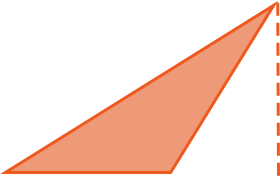### Step 1. Identify the base and height of the triangle.

Imagine the triangle is a mountain. The base must be flat and the height connects the base of the mountain to the peak.

CONCLUSION
Amazing work, look at you go! Thanks for checking out this lesson ☺️🙏. Where to next?Leave Feedback